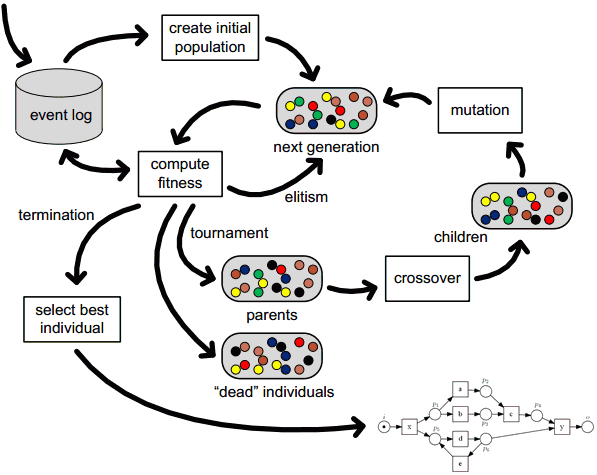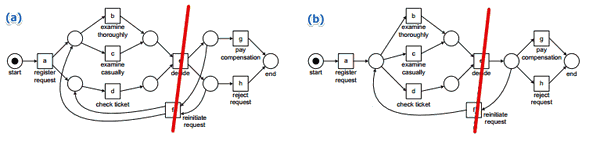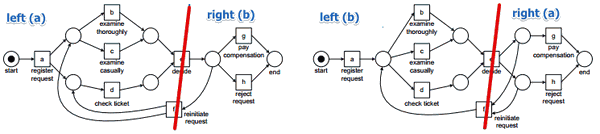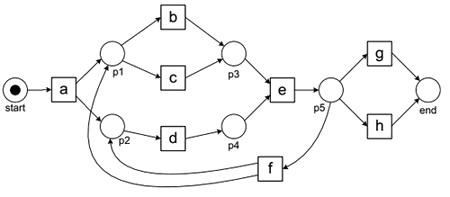# ML Wiki

## Genetic Process Miner

This is an algorithm from the family of Genetic Algorithms for mining business processes

• it's much more resilient to noise
• allows for incremental improvement
• can be combined with other approaches

### Overview• first we create the initial population
• at each step we compute fitness for all the instances of a population
• elitism - process of keeping the best ones
• then all "survived" instances are considered as "parents"
• cross-over - process of combining different petri nets (these petri-nets are already good solutions)
• mutation adding some random changes for diversifying

### Cross-Over

Cross-over

• a process of producing a "child" by two parental instances
• selecting parents
• completely at random
• using fitness to quicker converge to optima
• note that we should not always select only "the best" - we need diversity

How to create a petri net from two other petri nets?

• suppose we have two parental petri nets:
•• we may find some minimal cut, cut the parents and make children from then
• minimal cut is the minimal number of transitions to remove s.t. the net becomes completely disconnected
• the same as in the Graph Theory: Minimal Cut
• in this example:
• can cut in $(e, f)$ because in both cases
• from $e$ it goes to $g,h$ and on the left you have $a,b,c,d$
• so it's ideal
• we take $(e, f)$ out and get two disconnected components
• combine left and right parts of the petri nets to form children
•### Mutation

Examples of mutations:

• remove or add a place

### Parameters

So there are quite a few parameters that we have to provide:

• objective function we want to optimize (below)
• the way we do the cross-over
• how do you select parents?
• the way we do the mutations
• elitism - top 10%, certain threshold, etc
• how large the population should be
• how many generations you'll run before termination
• when we kill some instances?

Change one parameter

• $\Rightarrow$ different solution in the end

Also

• a lot of randomness is involved
• it may be computationally expensive

## Cost Function: Fitness

### Trace Level Fitness

Define trace-level fitness as:

• $\text{fitness}(\sigma, N) = \cfrac{1}{2} \left( 1 - \cfrac{m}{c} \right) + \cfrac{1}{2} \left( 1 - \cfrac{r}{p} \right)$
• $m$ - # of missing tokens
• $c$ - # of consumed tokens
• $r$ - # of tokens left over when something reaches the output place
• $p$ - # of produced tokens

### Example 1

Consider this example:

•• logtrace: $\sigma_1 = \langle abeg \rangle$
• $m = 0, c = 0, r = 0, p = 1$
• $p = 1$ because there's a token in the input place
• $a$ fires - it generates 2 tokens
• $p \leftarrow p + 2 = 3$
• $c \leftarrow c + 1 = 1$
• $b$ fires, produces one token, consumes one token
• $p \leftarrow p + 1 = 4$
• $c \leftarrow c + 1 = 2$
• $e$ needs to fire
• but it cannot: one token is missing, so we add it and fire $e$
• $p \leftarrow p + 1 = 5$
• $c \leftarrow c + 2 = 4$
• $m \leftarrow m + 1 = 1$
• $g$ fires and we're done
• $p \leftarrow p + 1 = 6$
• $c \leftarrow c + 1 = 5$
• but there's one remaining token that was left over after firing $a$
• $r \leftarrow r + 1 = 1$
• and finally we remove one token from the output place
• $c \leftarrow c + 1 = 6$
• $\text{fitness}(\sigma_1, N_1) = \cfrac{1}{2} \left( 1 - \cfrac{1}{6} \right) + \cfrac{1}{2} \left( 1 - \cfrac{1}{6} \right) = \cfrac{5}{6}$

### Example 2• logtrace: $\sigma_2 = \langle adceh \rangle$
• $p = 1, c = 0, m = 0, r = 0$
• $a$ fires, produces 1, consumes 1
• $p \leftarrow 2, c \leftarrow 1$
• $d$ needs to fire
• but there's no token in the place before $d$, so we need to put it there
• $m \leftarrow 1$
• $d$ fires: $p \leftarrow 3, c \leftarrow 2$
• $c$ fires
• $p \leftarrow 4, c \leftarrow 3$
• note that $c$ and $d$ were executed in order different from the order that the model can produce
• $e$ fires
• $p \leftarrow 5, c \leftarrow 4$
• $h$ fires
• $p \leftarrow 6, c \leftarrow 5$
• we're done
• one token is left: $r \leftarrow 1$
• taking the last token from $c$: $c \leftarrow 6$
• $\text{fitness}(\sigma_2, N_2) = \cfrac{1}{2} \left( 1 - \cfrac{1}{6} \right) + \cfrac{1}{2} \left( 1 - \cfrac{1}{6} \right) = \cfrac{5}{6}$

### Log Level Fitness

Log-level fitness is

• fitness, calculated for each trace and aggregated
• $\text{fitness}(L, N) = \cfrac{1}{2} \left( 1 - \cfrac{\sum_{\sigma \in L} L(\sigma) \times m_{N, \sigma}}{\sum_{\sigma \in L} L(\sigma) \times c_{N, \sigma} } \right) + \cfrac{1}{2} \left( 1 - \cfrac{\sum_{\sigma \in L} L(\sigma) \times r_{N, \sigma}}{\sum_{\sigma \in L} L(\sigma) \times p_{N, \sigma} } \right)$
• $L(\sigma)$ how many times the trace $\sigma$ occurred in log $L$